Скачать презентацию Econ 140 Binary Response Lecture 22 1

379a9301bfd00de5e2ef4ab454950978.ppt

• Количество слайдов: 21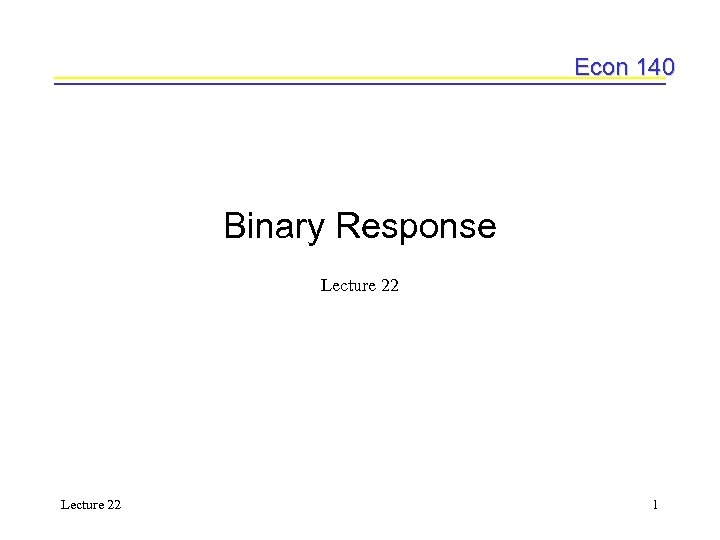Econ 140 Binary Response Lecture 22 1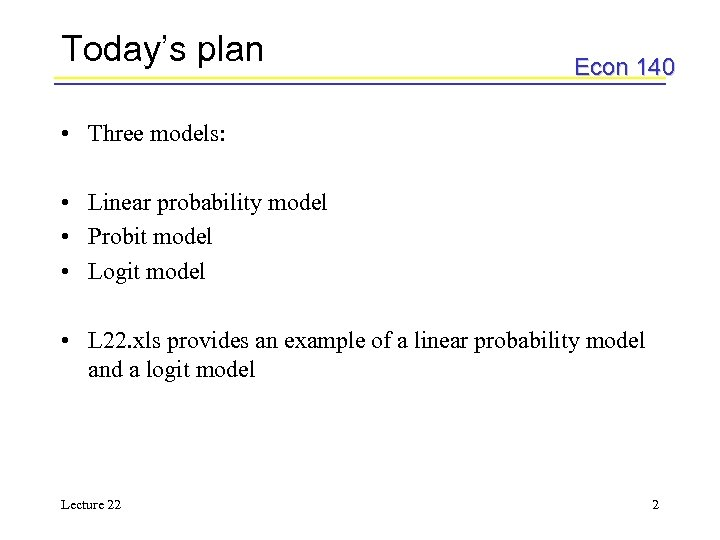Today’s plan Econ 140 • Three models: • Linear probability model • Probit model • Logit model • L 22. xls provides an example of a linear probability model and a logit model Lecture 22 2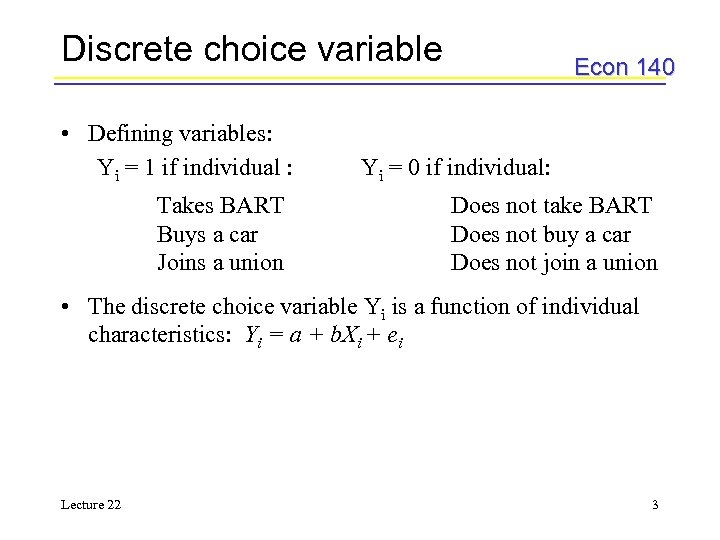Discrete choice variable • Defining variables: Yi = 1 if individual : Takes BART Buys a car Joins a union Econ 140 Yi = 0 if individual: Does not take BART Does not buy a car Does not join a union • The discrete choice variable Yi is a function of individual characteristics: Yi = a + b. Xi + ei Lecture 22 3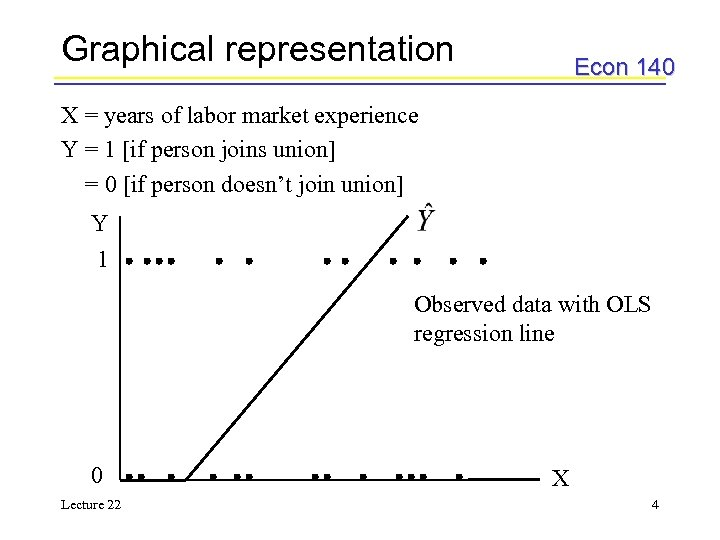Graphical representation Econ 140 X = years of labor market experience Y = 1 [if person joins union] = 0 [if person doesn’t join union] Y 1 Observed data with OLS regression line 0 Lecture 22 X 4Linear probability model Econ 140 • The OLS regression line in the previous slide is called the linear probability model – predicting the probability that an individual will join a union given their years of labor market experience • Using the linear probability model, we estimate the equation: – using Lecture 22 we can predict the probability 5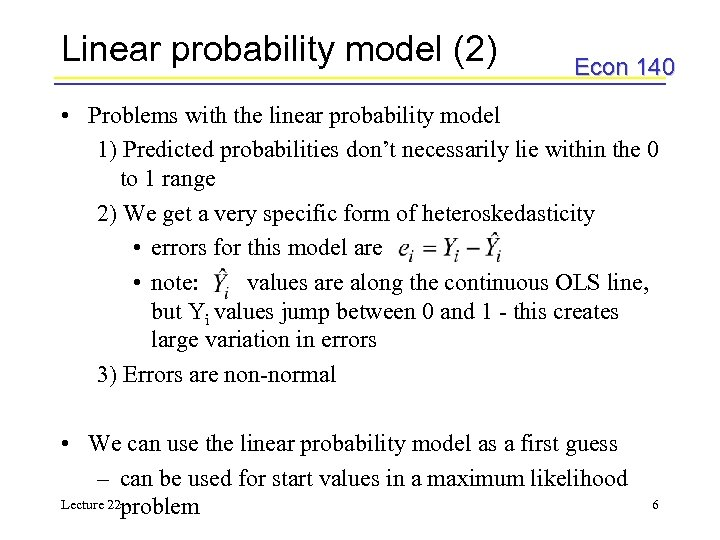Linear probability model (2) Econ 140 • Problems with the linear probability model 1) Predicted probabilities don’t necessarily lie within the 0 to 1 range 2) We get a very specific form of heteroskedasticity • errors for this model are • note: values are along the continuous OLS line, but Yi values jump between 0 and 1 - this creates large variation in errors 3) Errors are non-normal • We can use the linear probability model as a first guess – can be used for start values in a maximum likelihood Lecture 22 problem 6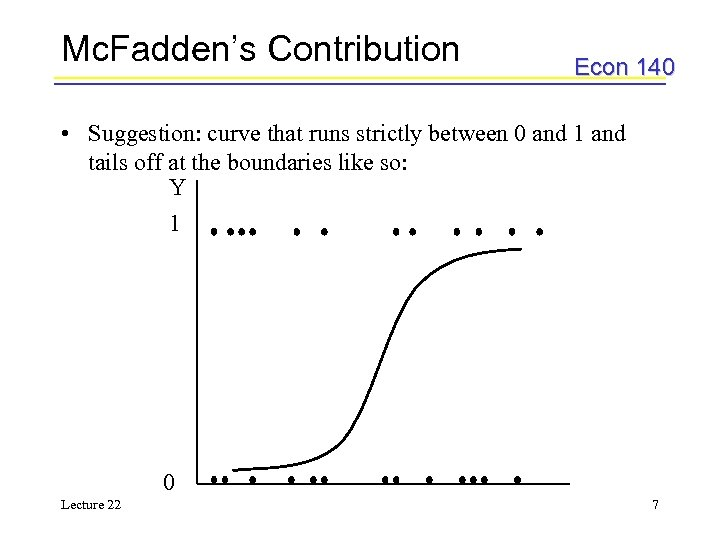Mc. Fadden’s Contribution Econ 140 • Suggestion: curve that runs strictly between 0 and 1 and tails off at the boundaries like so: Y 1 0 Lecture 22 7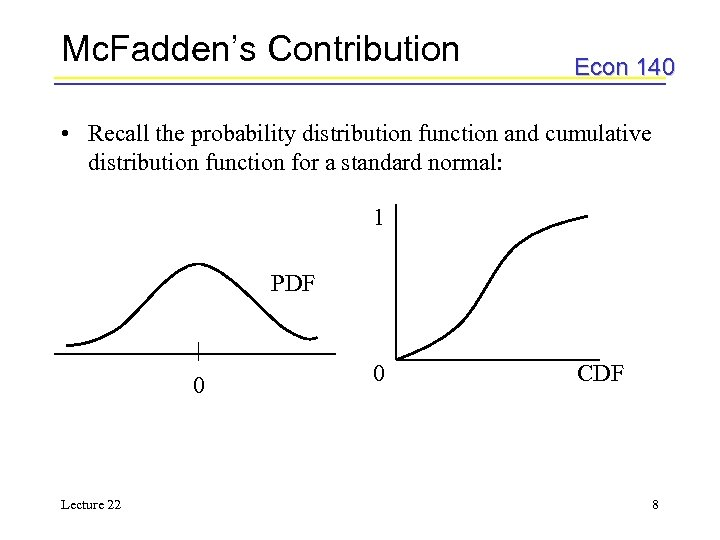Mc. Fadden’s Contribution Econ 140 • Recall the probability distribution function and cumulative distribution function for a standard normal: 1 PDF 0 Lecture 22 0 CDF 8Probit model Econ 140 • For the standard normal, we have the probit model using the PDF • The density function for the normal is: where Z = a + b. X • For the probit model, we want to find Lecture 22 9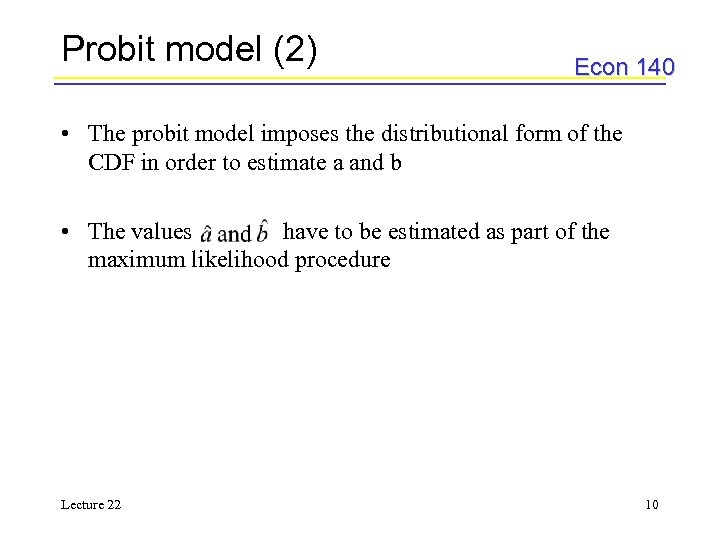Probit model (2) Econ 140 • The probit model imposes the distributional form of the CDF in order to estimate a and b • The values have to be estimated as part of the maximum likelihood procedure Lecture 22 10Logit model Econ 140 • The logit model uses the logistic distribution Density: 1 Cumulative: Standard normal F(Z) Logistic G(Z) 0 Lecture 22 11Maximum likelihood Econ 140 • Alternative estimation that assumes you know the form of the population • Using maximum likelihood, we will be specifying the model as part of the distribution Lecture 22 12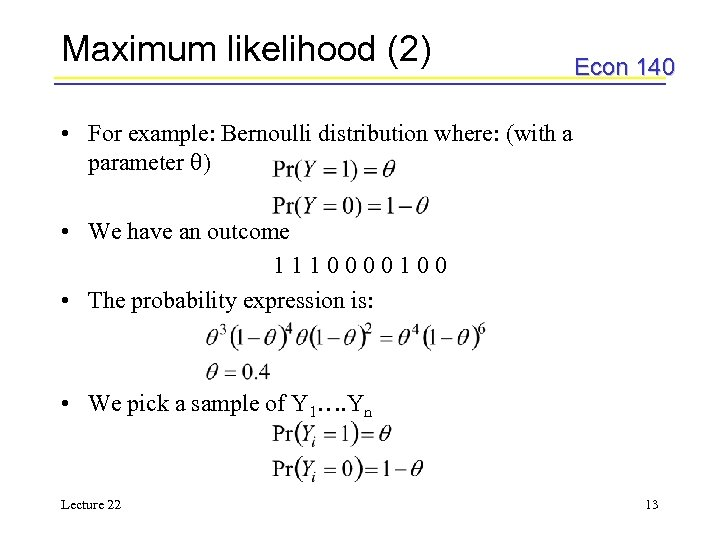Maximum likelihood (2) Econ 140 • For example: Bernoulli distribution where: (with a parameter ) • We have an outcome 1110000100 • The probability expression is: • We pick a sample of Y 1…. Yn Lecture 22 13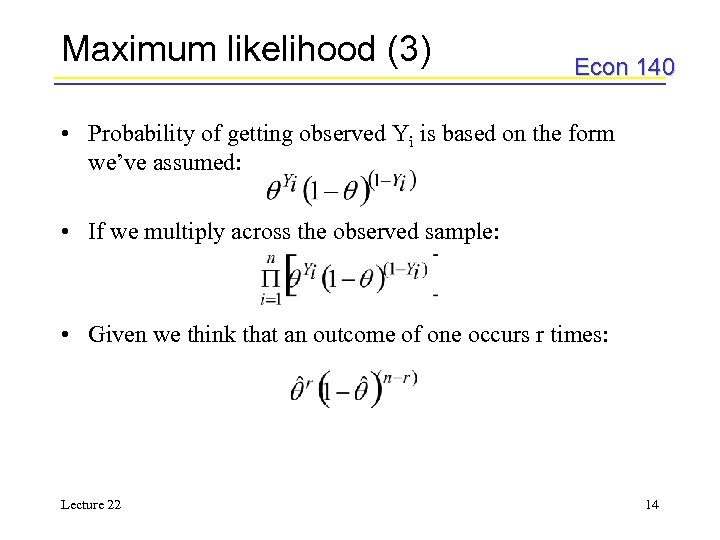Maximum likelihood (3) Econ 140 • Probability of getting observed Yi is based on the form we’ve assumed: • If we multiply across the observed sample: • Given we think that an outcome of one occurs r times: Lecture 22 14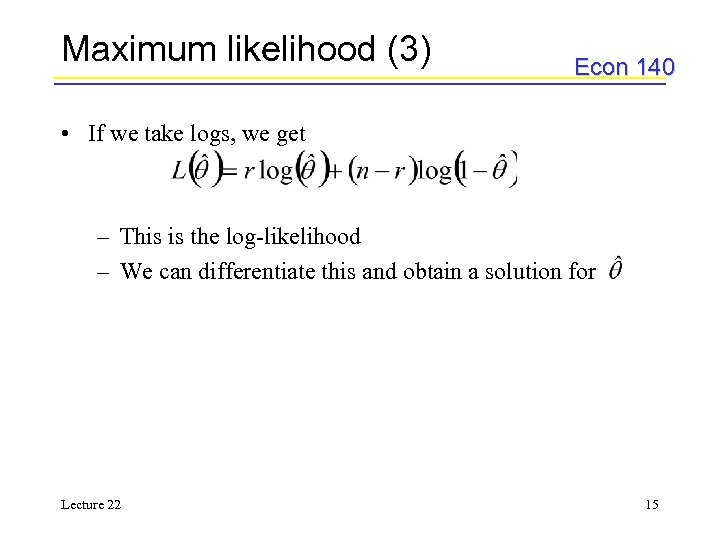Maximum likelihood (3) Econ 140 • If we take logs, we get – This is the log-likelihood – We can differentiate this and obtain a solution for Lecture 22 15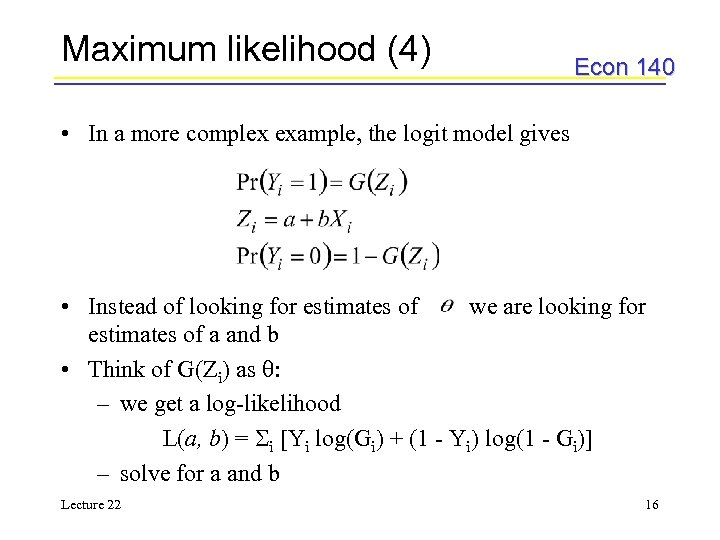Maximum likelihood (4) Econ 140 • In a more complex example, the logit model gives • Instead of looking for estimates of we are looking for estimates of a and b • Think of G(Zi) as : – we get a log-likelihood L(a, b) = Si [Yi log(Gi) + (1 - Yi) log(1 - Gi)] – solve for a and b Lecture 22 16Example Econ 140 • Data on union membership and years of labor market experience (L 22. xls) • To build the maximum likelihood form, we can think of: – intercept: a – coefficient on experience : b • There are three columns – Predicted value Z – Estimated probability – Estimated likelihood as given by the model • The Solver from the Tools menu calculates estimates of a and b Lecture 22 17Example (2) Econ 140 • How the solver works: • Defining a and b using start values • Choose start values of a and b equal to zero • Define our model: Z = a + b. X • Define the predictive possibilities: • Define the log-likelihood and sum it – Can use Solver to change the values on a and b Lecture 22 18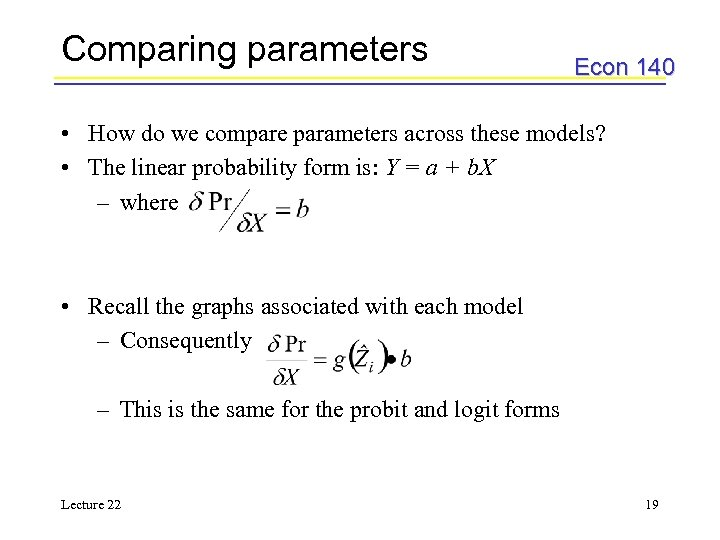Comparing parameters Econ 140 • How do we compare parameters across these models? • The linear probability form is: Y = a + b. X – where • Recall the graphs associated with each model – Consequently – This is the same for the probit and logit forms Lecture 22 19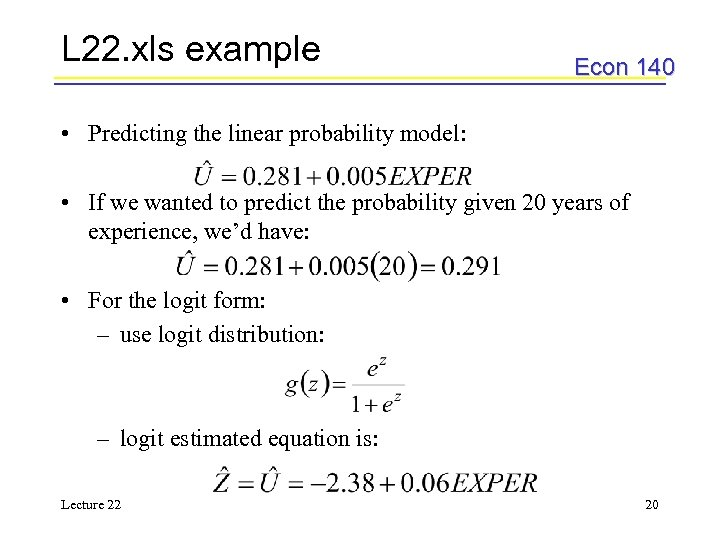L 22. xls example Econ 140 • Predicting the linear probability model: • If we wanted to predict the probability given 20 years of experience, we’d have: • For the logit form: – use logit distribution: – logit estimated equation is: Lecture 22 20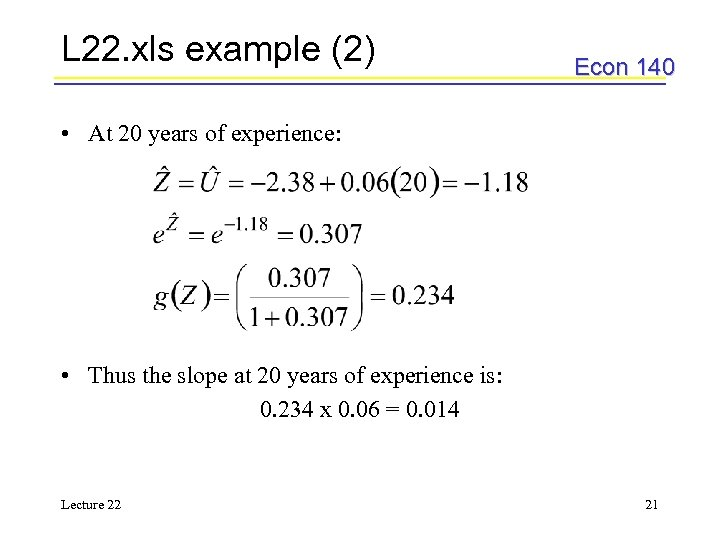L 22. xls example (2) Econ 140 • At 20 years of experience: • Thus the slope at 20 years of experience is: 0. 234 x 0. 06 = 0. 014 Lecture 22 21